Test: Boolean Algebra & Minimization Techniques - 1

# Test: Boolean Algebra & Minimization Techniques - 1

Test Description

## 10 Questions MCQ Test | Test: Boolean Algebra & Minimization Techniques - 1

Test: Boolean Algebra & Minimization Techniques - 1 for Electronics and Communication Engineering (ECE) 2023 is part of Electronics and Communication Engineering (ECE) preparation. The Test: Boolean Algebra & Minimization Techniques - 1 questions and answers have been prepared according to the Electronics and Communication Engineering (ECE) exam syllabus.The Test: Boolean Algebra & Minimization Techniques - 1 MCQs are made for Electronics and Communication Engineering (ECE) 2023 Exam. Find important definitions, questions, notes, meanings, examples, exercises, MCQs and online tests for Test: Boolean Algebra & Minimization Techniques - 1 below.
Solutions of Test: Boolean Algebra & Minimization Techniques - 1 questions in English are available as part of our course for Electronics and Communication Engineering (ECE) & Test: Boolean Algebra & Minimization Techniques - 1 solutions in Hindi for Electronics and Communication Engineering (ECE) course. Download more important topics, notes, lectures and mock test series for Electronics and Communication Engineering (ECE) Exam by signing up for free. Attempt Test: Boolean Algebra & Minimization Techniques - 1 | 10 questions in 30 minutes | Mock test for Electronics and Communication Engineering (ECE) preparation | Free important questions MCQ to study for Electronics and Communication Engineering (ECE) Exam | Download free PDF with solutions
 1 Crore+ students have signed up on EduRev. Have you?
Test: Boolean Algebra & Minimization Techniques - 1 - Question 1

### Three Boolean operators are

Detailed Solution for Test: Boolean Algebra & Minimization Techniques - 1 - Question 1

The three Boolean operators are NOT, OR, AND, These are also called basic gates for digital electronics.

Test: Boolean Algebra & Minimization Techniques - 1 - Question 2

### The only function of a NOT gate is to

Detailed Solution for Test: Boolean Algebra & Minimization Techniques - 1 - Question 2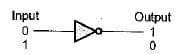NOT gate is used to invert the input signal.

Test: Boolean Algebra & Minimization Techniques - 1 - Question 3

### Negative logic in 'a logic circuit is one in which

Detailed Solution for Test: Boolean Algebra & Minimization Techniques - 1 - Question 3

In negative logic:

• logic 0 = Some positive voltage
• logic 1 = Zero voltage

or

• logic 0 = Some higher voltage level (i.e. + 5 V,)
• logic 1 = Some lower voltage level (i.e. + 2 V)
Test: Boolean Algebra & Minimization Techniques - 1 - Question 4

Which of the following Boolean Expressions is not true?

Detailed Solution for Test: Boolean Algebra & Minimization Techniques - 1 - Question 4

A + 1 = 1 (Always)
Hence,  option (c) is not correct.

Test: Boolean Algebra & Minimization Techniques - 1 - Question 5

The simplified form of the Boolean expression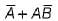is

Detailed Solution for Test: Boolean Algebra & Minimization Techniques - 1 - Question 5

Using distribution theorem, we have: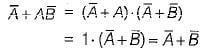Test: Boolean Algebra & Minimization Techniques - 1 - Question 6

The Boolean expression: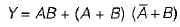may be simplified as

Detailed Solution for Test: Boolean Algebra & Minimization Techniques - 1 - Question 6

Given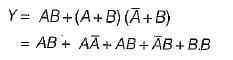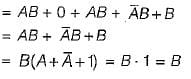Test: Boolean Algebra & Minimization Techniques - 1 - Question 7

The complement form of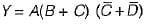is

Detailed Solution for Test: Boolean Algebra & Minimization Techniques - 1 - Question 7

Given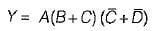∴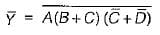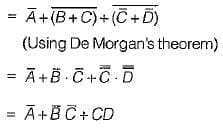Test: Boolean Algebra & Minimization Techniques - 1 - Question 8

The POS form of expression is suitable for circuit using:

Detailed Solution for Test: Boolean Algebra & Minimization Techniques - 1 - Question 8

POS form (Product of Sum form) of expression is suitable for circuit using NOR gates.

Test: Boolean Algebra & Minimization Techniques - 1 - Question 9

Which of the following is/are the correct reason for using Gray code in K-maps?

Test: Boolean Algebra & Minimization Techniques - 1 - Question 10

When eight adjacent 1 's are grouped horizontally or vertically the group so found is called

Detailed Solution for Test: Boolean Algebra & Minimization Techniques - 1 - Question 10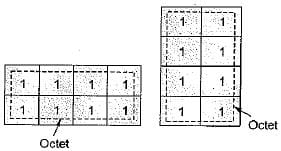Information about Test: Boolean Algebra & Minimization Techniques - 1 Page
In this test you can find the Exam questions for Test: Boolean Algebra & Minimization Techniques - 1 solved & explained in the simplest way possible. Besides giving Questions and answers for Test: Boolean Algebra & Minimization Techniques - 1, EduRev gives you an ample number of Online tests for practice(Scan QR code)